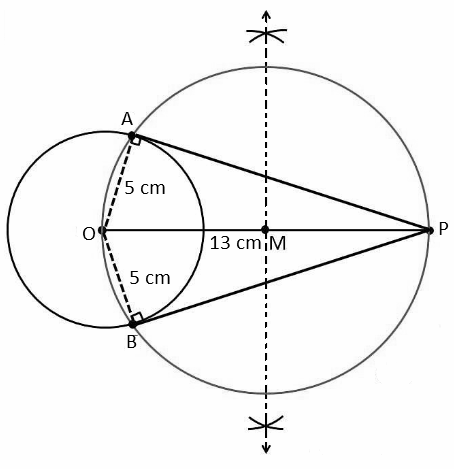# RBSE Maths Class 10 Chapter 14: Constructions Important Questions and Solutions

RBSE Class 10 Maths Chapter 14 – Constructions Important questions and solutions are given here. All these questions are provided, as per the new guidelines of the board to help students get updated with the new syllabus. The RBSE Class 10 important questions and solutions given at BYJU’S contain all the important concepts, which will be useful for the students for their higher classes.

Chapter 14 of RBSE Class 10 has only two exercises which have questions on the construction of tangents to the circle, incircle, circumcircle of triangles. After practising all these important questions, students will be able to understand how to deal with constructions related to various theorems, which have been discussed in their earlier classes.

### RBSE Maths Chapter 14: Exercise 14.1 Textbook Important Questions and Solutions

Question 1: Draw a line segment of length 6.7 cm and divide it into the ratio of 2 : 3 internally.

Solution:

Steps of construction:

(i) Draw a line segment AB of length 6.7 cm.

(ii) Draw a ray AX, making an acute angle with AB.

(iii) Mark 5 points (i.e. 2 + 3 = 5) A1, A2, A3, A4 and A5 on AX such that AA1 = A1A2 = A2A3 = A3A4 = A4A5.

(iv) Join BA5.

(v) Through the point A2, draw a line parallel to BA5 by making an angle equal to ∠AA5B such that it intersects AB at C.

Hence, AC : CB = 2 : 3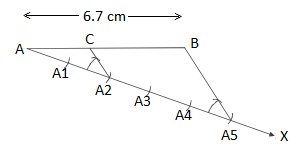Question 2: Draw a line segment AB of length 8.3 cm. Find a point C on AB such that AC = (1/3)AB and also verify it.

Solution:

Steps of construction:

(i) Draw a line segment AB of length 6.7 cm.

(ii) Draw a ray AX, making an acute angle with AB.

(iii) Mark 3 points A1, A2, and A3 on AX such that AA1 = A1A2 = A2A3.

(iv) Join BA3.

(v) Through the point A1, draw a line parallel to BA3 by making an angle equal to ∠AA3B such that it intersects AB at C.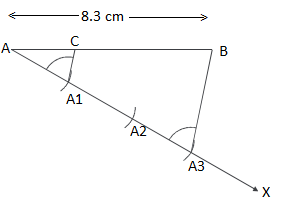Verification:

BC/AC = A1A3/AA1

(BC + AC) + 1 = (A1A3/AA1) + 1

(BC + AC)/AC = (AA1 + A1A3)/AA1

AB/AC = (AA1 + A1A3)/AA1 = 3/1

⇒ AC/AB = 1/3

⇒ AC = (1/2) AB

Question 3: Take a point P on the circle of radius 2.8 cm and draw a tangent to the circle at P.

Solution:

Steps of construction:

(i) Draw a circle with the centre O and radius 2.8 cm.

(ii) Take a point P on the circle and join OP.

(iii) Draw a perpendicular at P so that ∠OPA = 90° and extend the perpendicular on both sides upto A and B.

Hence, APB is the required tangent.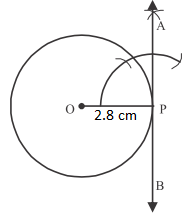Question 4: Draw the tangents to both the ends of the diameter of the circle of radius 3 cm. Will the tangents intersect each other? If yes, justify your answer.

Solution:

Steps of construction:

(i) Draw a circle with the centre O and radius 3 cm.

(ii) Draw a diameter POQ.

(iii) Draw a perpendicular at P so that ∠OPA = 90° and extend the perpendicular on both sides upto A and B.

(iii) Draw a perpendicular at Q so that ∠OQA’ = 90° and extend the perpendicular on both sides upto A’ and B’.

Hence, APB and A’QB’ are the required tangents.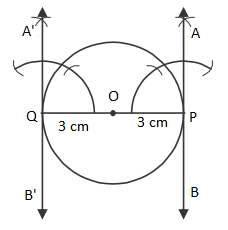Here, tangents are parallel to each other and hence they will never intersect each other.

Question 5: Draw a chord of length 2.3 cm in a circle of radius 3.1 cm and draw the tangent to its both ends.

Solution:

Steps of construction:

(i) Draw a circle with centre O and radius 3.1 cm.

(ii) Taking a point A on the circumference of the circle as a center and radius 2.3 cm, draw an arc to intersect the circle at B.

(iii) By joining PQ, chord PQ will obtain.

(iv) Draw perpendiculars AP and BR on OA and OB (i.e. on radius), respectively.

(v) Produce AP upto Q and BR upto S.

Hence, PQ and RS are the required tangents to the circle.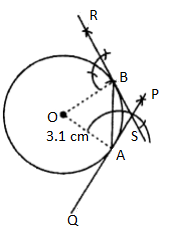Question 6: Draw a tangent to a circle of radius 2.7 cm.

Solution:

Steps of construction:

(i) Draw a circle with the centre O and radius 2.7 cm.

(ii) Take a point P on the circle and join OP.

(iii) Draw a perpendicular at P so that ∠OPA = 90° and extend the perpendicular on both sides upto A and B.

Hence, APB is the required tangent.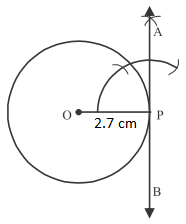Question 7: Draw a circle with centre O and radius 2.4 cm. Draw two radii OA and OB such that the angle between them is 60°. Draw the tangent at A and B to intersect each other at T. Measure the angle ATB.

Solution:

Steps of construction:

(i) Draw a circle with centre O radius 2.4 cm.

(ii) At center O, draw two radii OA and OB such that ∠AOB = 60°.

(iii) At point A of OA draw a perpendicular.

(iv) At point B of OB draw another perpendicular, which intersects the previously drawn line at T.

Hence, AT and BT are the required tangents also, the measurement of ∠ATB is 120°.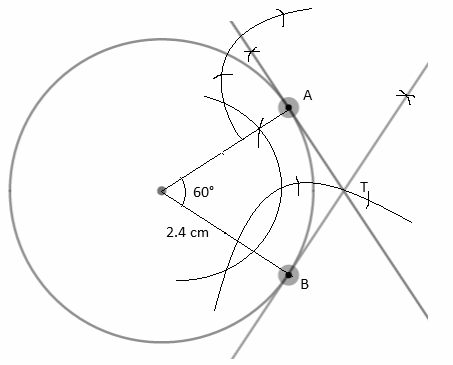Question 8: Draw two tangents to the circle of radius 13.2 cm such that the angle between them is 70°.

Solution:

Given,

Angle between two tangent = 70°

Angle made by the line segments joining points of contact and the centre of the circle = 180° – 70° = 110°

Steps of construction:

(i) Draw a circle with center O and radius 13.2 cm.

(ii) At center O, draw two radii OA and OB such that ∠AOB = 110°.

(iii) Draw perpendiculars at A and B of OA and OB respectively such that intersect each other at point P.

Hence, AP and BP are the required tangents and ∠APB = 70°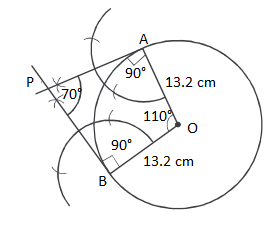Question 9: Draw a circle of radius 3 cm. Construct a pair of tangents from an exterior point 5 cm away from its centre.

Solution:

Steps of construction:

(i) Draw a circle with center O and radius 3 cm.

(ii) Take a point P at a distance of 5 cm from the centre of the circle.

(iii) Join OP and draw its perpendicular bisector which intersects OP at M.

(iv) Taking M as the centre and radius PM, draw another circle which intersects the given circle at A and B.

(v) Join PA and PB using rays.

Hence PA and PB are the required tangents.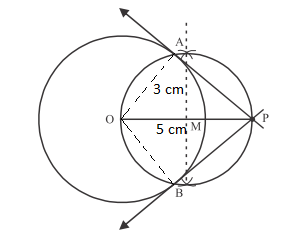Question 10: The distance between the centres of two circles is 8 cm. Radius of one circle is 3 cm and that of the other is 4 cm. How many common tangents can be drawn on these circles and construct two direct common tangents?

Solution:

Let r1 = 3 cm and r2 = 4 cm

Let C1 and C2 be the centres of circles with radius r1 and r2 respectively.

C1C2 = 8 cm

C1C2 > r1 + r2

Thus, four common tangents (two direct and two transversal) can be drawn to these two circles.

Steps of construction:

(i) Draw a line segment C1C2 of length 8 cm.

(ii) Taking C1 as the centre and radius 3 cm draw a circle.

(iii) Taking C2 as the centre and radius 4 cm draw another circle.

(iv) Draw the perpendicular bisector of C1C2 which intersects C1C2 at M.

(v) Taking M as center and radius equal to MC1 or MC2 draw another circle.

(vi) Taking C2 as the centre and radius 1 cm (r2 – r1 = 4 – 3 = 1) draw a circle which intersects the circle center M at N1 and N2.

(vii) Join C2N1 and C2N2 and produce to intersect the given circle at B and Q.

(viii) Draw C1A || C2B and C1P || C2Q.

(ix) Join AB and PQ.

Hence, AB and PQ are the required tangents.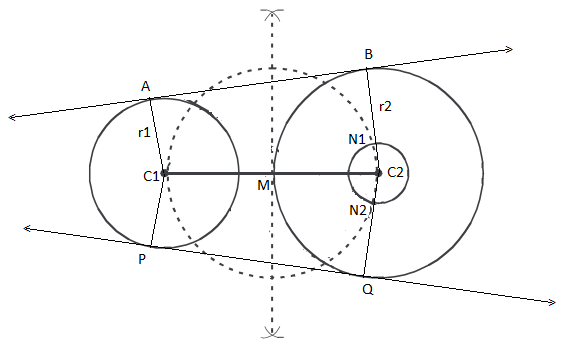Question 11: Construct a common transversal tangents of the circles whose radii are 1.7 cm and 2.8 cm respectively and centres are 6 cm apart.

Solution:

Let r1 and r2 be the radii of circles with centres C1 and C2.

r1 = 1.7 cm, r2 = 2.8 cm

C1C2 = 6 cm

r1 + r2 = 1.7 + 2.8 = 4.5 < C1C2

Steps of construction:

(i) Draw a line segment C1C2 of the length of 6 cm.

(ii) Taking C1 as centre and radius 1.7 cm draw a circle.

(iii) Taking C2 as centre and radius 2.8 cm draw another circle.

(iv) Draw the perpendicular bisector of C1C2 which intersects it at M.

(v) Taking M as the centre and radius equal to MC1 or MC2 draw another circle.

(vi) Taking C1 as the centre and the radius equal to the sum of two radii 1.7 + 2.8 = 4.5 cm draw a circle which intersects the circle with diameter C1C2 at P and Q.

(vii) Join PC2 and PC1 which intersect the circle radius 1.7 cm at A.

(viii) Join AB.

Hence, AB is the required indirect common tangent.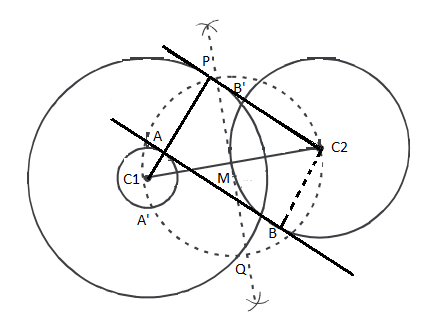### RBSE Maths Chapter 14: Exercise 14.2 Textbook Important Questions and Solutions

Question 12: Find the true or false in the following statements and justify your answer, if possible.

(i) Circumference and incircle of an equilateral triangle can be drawn from the same centre.

(ii) All three sides of a triangle touch its incircle.

(iii) If the triangle is obtuse angled, its circumference will fall at one of its sides.

(iv) The circumference of the triangle lies inside, if the triangle is an acute triangle.

(v) The construction of an incircle is being done by obtaining the point of intersection of two perpendiculars of sides and bisector of two angles.

Solution:

(i) True – Circumcentre, incentre and orthocentre of an equilateral triangle coincide.

(ii) True – An incircle is drawn with the radius equal to length of perpendicular to a side from incentre.

(iii) False – A right triangle has its circumcentre on its hypotenuse.

(iv) True – Circumference of the triangle lies inside, if the triangle is an acute triangle.

(v) False – Constructing an incircle of a triangle can be done by bisecting its angle not its sides

Question 13: Construct an incircle of an equilateral triangle with side 4.6 cm. Does its incentre and circumcentre coincide? Justify your answer.

Solution:

Steps of construction:

(i) Draw a line segment BC of length 4.6 cm

(ii) Taking B as center and with 4.6 cm as radius draw an arc.

(iii) Taking C as center and with 4.6 cm as radius draw another arc, which intersect each other at A.

(iv) Join AB and AC hence, ABC is the required equilateral triangle.

(v) Draw the bisectors of ∠ABC and ∠ACB, which intersect each other at O.

(vi) Draw OP ⊥ BC from O.

(vii) Taking O as the centre and radius equal to OP, draw a circle which touches the three sides of triangle ABC.

Hence, this is required incircle of an equilateral triangle ABC.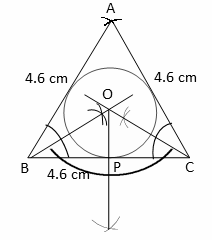All the sides and angles of an equilateral triangle are equal so its circumcentre and incentre coincide.

Question 14: Construct an incircle of a triangle with AB = 4.6 cm and AC = 4.2 cm and ∠A = 90°.

Solution:

Steps of construction:

(i) Draw a line segment AB of length 4.6 cm as the base.

(ii) Construct an angle of 90° at A and mark a point C at a distance of 4.2 from A.

(3) Join BC and ΔABC is the right triangle.

(4) Draw the bisectors of ∠A and ∠B which intersect each other at O.

(5) Draw OM ⊥ AB from O.

(6) Taking O as the centre and with radius OD, draw a circle which touches three sides of triangle ABC.

Hence, thus is the required incircle of right triangle ABC.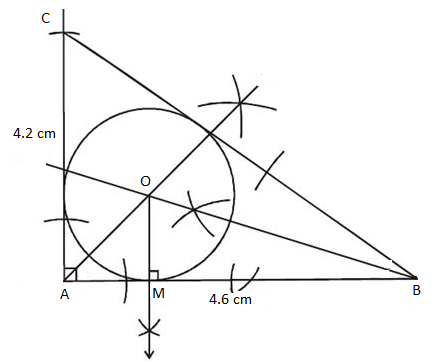Question 15: Draw a circumcircle of a triangle with sides respectively 5, 12 and 13 cm. Why does its circumference fall at the side of length 12 cm?

Solution:

Steps of construction:

(i) Draw a line segment BC of length 13 cm.

(ii) Taking B as the centre and with radius 12 cm, draw an arc.

(iii) Taking C as the centre and with radius 5 cm, draw another arc which intersects the previous arc at A.

(iv) Join AB and AC hence triangle ABC will obtain.

(v) Draw the perpendiculars of sides AB and AC such that they intersect each other at O.

(vi) Taking O as the centre and radius equal to OA or OB or OC, draw a circle.

(vii) The circle passing through all the three vertices of the triangle ABC.

Hence, this is the required circumcircle of triangle ABC.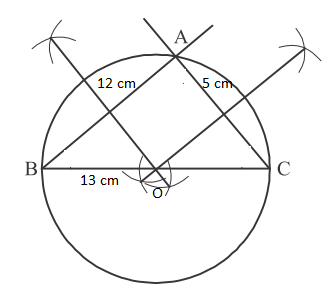Circumcenter lies on the side of 13 cm but not on the side of 12 cm since the obtained triangle is right angle.

Question 16: Draw a circumcircle of a triangle whose sides are 5 cm, 4.5 cm and 7 cm. Where and why does its circumcentre lie?

Solution:

Steps of construction:

(i) Draw a line segment of length 5 cm as the base.

(ii) Taking A as the centre and radius 4.5 cm, draw an arc.

(iii) Taking B as the centre and radius 7 cm, draw another arc which intersects the previous arc at C.

(iv) Join AC and BC.

(v) Hence, triangle ABC obtained is an obtuse angled triangle.

(vi) Draw the perpendiculars of AB and BC which intersect each other at O outside the triangle.

(vii) Taking O as the centre and radius equal to OA, draw a circle which passes through the vertices of triangle ABC.

Hence, this is the required circumcircle of the triangle.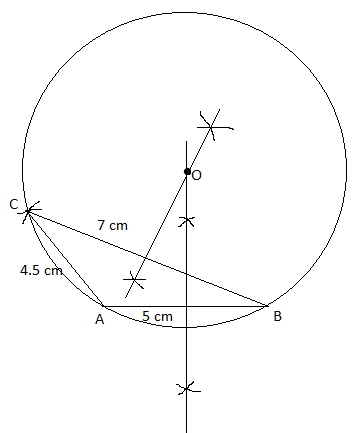Question 17: Construct a triangle ABC in which AB = 6 cm, BC = 4 cm and ∠B = 120°. Also, construct an incircle of this triangle.

Solution:

Steps of construction:

(i) Draw a line segment of BC of length 4 cm as the base.

(ii) Construct an angle of 120° at B and by taking B as the centre and radius 6 cm, draw an arc which meets the angle line at A.

(iii) Join AC and the triangle ABC will obtain.

(iv) Draw the angle bisectors of B and C which intersect each other at O.

(v) Draw a perpendicular OM on AC from O.

(vi) Taking O as the centre and OM as the radius, draw a circle which touches all three sides of the triangle.

Hence, this is the required incircle for triangle ABC.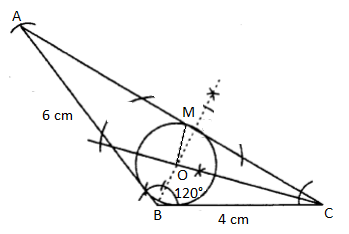### RBSE Maths Chapter 14: Additional Important Questions and Solutions

Question 1: Construct an incircle of an equilateral triangle with side 5 cm.

Solution:

Steps of construction:

(i) Draw a line segment BC of length 5 cm

(ii) Taking B as center and with 5 cm as radius draw an arc.

(iii) Taking C as center and with 5 cm as radius draw another arc, which intersect each other at A.

(iv) Join AB and AC hence, ABC is the required equilateral triangle.

(v) Draw the bisectors of ∠ABC and ∠ACB, which intersect each other at O.

(vi) Draw OP ⊥ BC from O.

(vii) Taking O as the centre and radius equal to OP, draw a circle which touches the three sides of triangle ABC.

Hence, this is required incircle of an equilateral triangle ABC.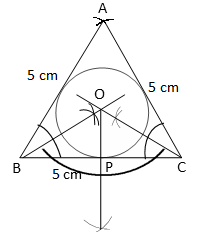Question 2: Draw two tangents PA and PB from an external point P to a circle of radius 4 cm where angle between PA and PB is 65°.

Solution:

Given,

Angle between two tangent = 65°

Angle made by the line segments joining points of contact and the centre of the circle = 180° – 65° = 115°

Steps of construction:

(i) Draw a circle with center O and radius 4 cm.

(ii) At center O, draw two radii OA and OB such that ∠AOB = 115°.

(iii) Draw perpendiculars at A and B of OA and OB, respectively such that they intersect each other at point P.

Hence, PA and PB are the required tangents and ∠APB = 65°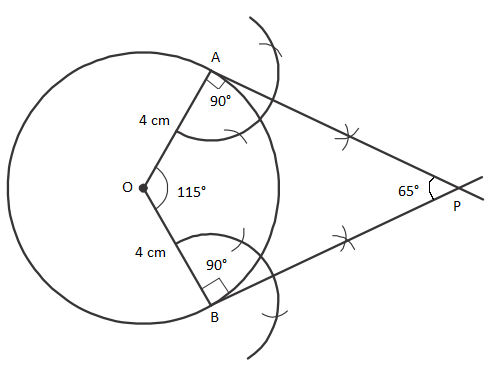Question 3: Draw a circle of radius 5 cm. From a point 13 cm away from its centre, construct the pair of tangents to the circle and measure their length. Also, verify the measurement by actual calculation.

Solution: